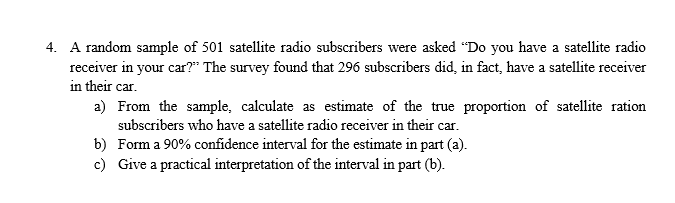# A random sample of 501 satellite radio subscribers were asked "Do you have a satellite radioreceiver in your car?" The survey found that 296 subscribers did, in fact, have a satellite receiverin their car.4.a) From the sample, calculate as estimate of the true proportion of satellite rationsubscribers who have a satellite radio receiver in their car.Form a 90% confidence interval for the estimate in part (a).Give a practical interpretation of the interval in part (b).b)c)

Question
72 viewshelp_outlineImage TranscriptioncloseA random sample of 501 satellite radio subscribers were asked "Do you have a satellite radio receiver in your car?" The survey found that 296 subscribers did, in fact, have a satellite receiver in their car. 4. a) From the sample, calculate as estimate of the true proportion of satellite ration subscribers who have a satellite radio receiver in their car. Form a 90% confidence interval for the estimate in part (a). Give a practical interpretation of the interval in part (b). b) c) fullscreen
check_circle

Step 1

True proportion estimate will be given by the ratio of success/favorable outcomes and the total sample size.

True proportion (in this case ) is calculated as shown below.

Step 2

Let sample proportion be denoted by p-hat.  Confidence interval can be calculated using the formula shown below as long as there are atleast 5 successes (favorable outcomes) and 5 failures (unfavorabl outcomes) in the sample.

Step 3

Confidence interval: (0.555, 0.627)  when confidence level is 90%

This would mea...

### Want to see the full answer?

See Solution

#### Want to see this answer and more?

Solutions are written by subject experts who are available 24/7. Questions are typically answered within 1 hour.*

See Solution
*Response times may vary by subject and question.
Tagged in

### Measures